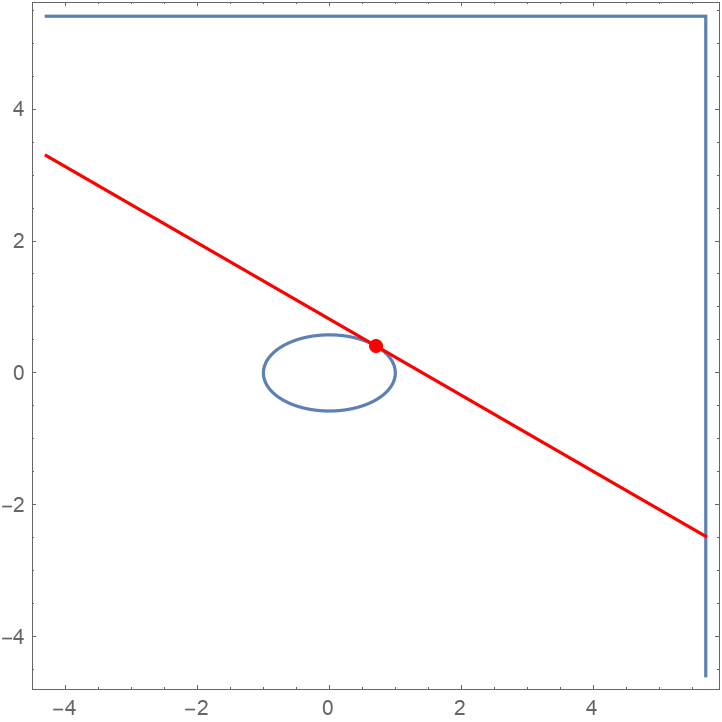Function Repository Resource:

TangentLine

Compute properties of the tangent line to a curve at a given point

Contributed by: Wolfram|Alpha Math Team
 ResourceFunction["TangentLine"][expr,{x,a},{y,b}] gives an association of properties of the tangent line to expr, viewed as an equation in x and y, at the point {x,y}={a,b}. ResourceFunction["TangentLine"][expr,{x,a},{y,b},prop] returns the value of the tangent line property prop. ResourceFunction["TangentLine"][expr,{x,a},y,…] returns information relating to one, among possibly several, of the tangent lines to expr at x=a.

Details and Options

Allowed values of prop are: "SlopeInterceptEquation", "StandardFormEquation", "PointSlopeEquation", "HorizontalIntercept", "VerticalIntercept", "Plot" and All (default).
If expr does not have head Equal, then expr is treated as an expression defining y in terms of x. In other words, ResourceFunction["TangentLine"][expr,{x,a},y,] is equivaluent to ResourceFunction["TangentLine"][y==expr,{x,a},y,] if expr itself has a head other than Equal.
If no value for y at the intersection point is given, one is inferred. For expressions that are multivalued at x=a, information on only one of potentially several tangent lines is returned unless y is also specified.

Examples

Basic Examples

Compute the slope-intercept equation of the tangent line to a curve at a given point:

 In:=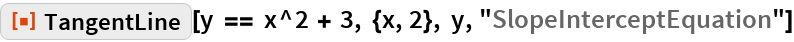Out=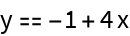Visualize this result:

 In:=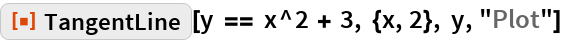Out=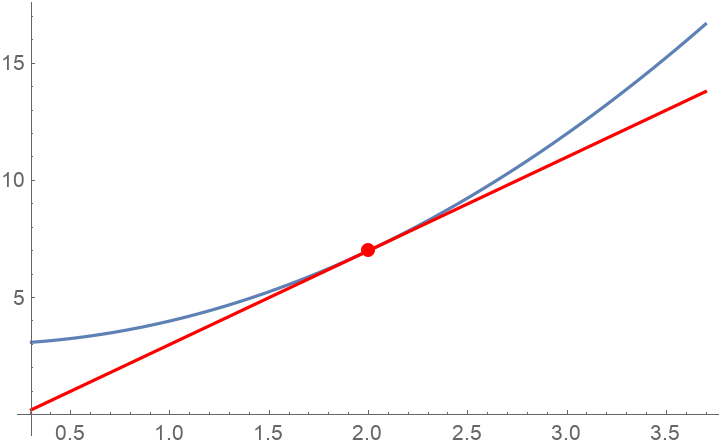Compute the slope of this tangent line:

 In:=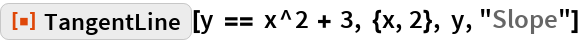Out=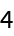Compute the horizontal intercept of this tangent line:

 In:=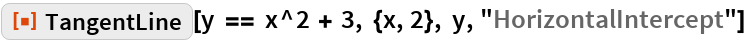Out=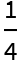Get the standard-form equation of this tangent line:

 In:=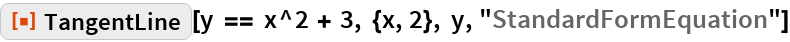Out=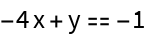Get an association of properties of a tangent line to a curve:

 In:=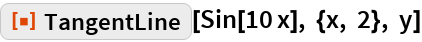Out=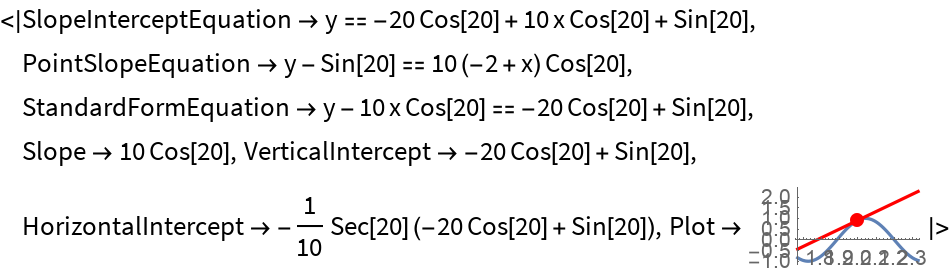Get just the point-slope equation of this tangent line:

 In:=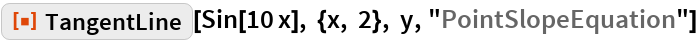Out=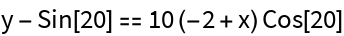Scope

The first argument to TangentLine can be an implicit definition of a curve:

 In:=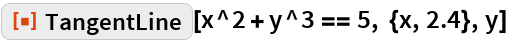Out=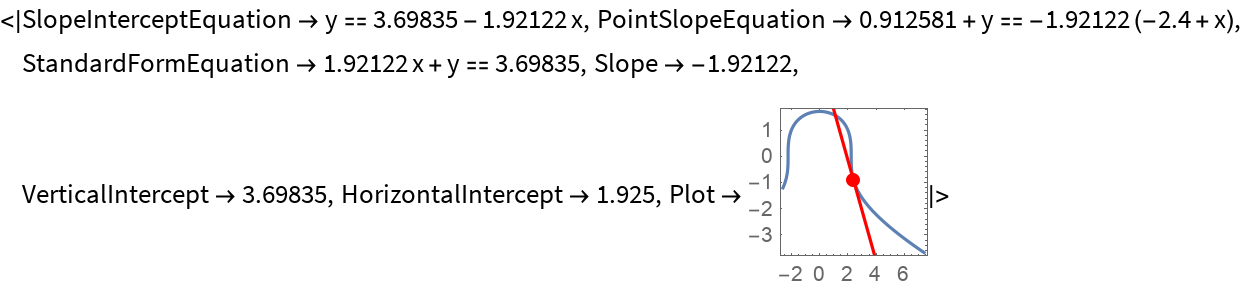Properties and Relations

If a tangent line coincides with a coordinate axis, its intercept with that axis is the set of real numbers:

 In:=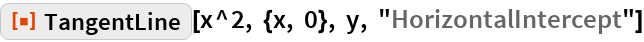Out=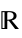If a tangent line is parallel to, but does not coincide with, a coordinate axis, its intercept with that axis is None:

 In:=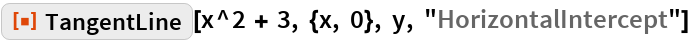Out=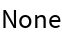Requesting tangent line information about a point that is not on the curve will result in an error message:

 In:=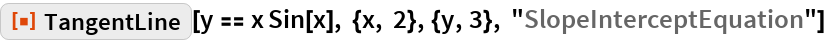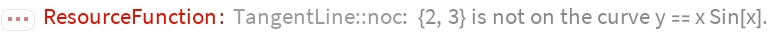Out=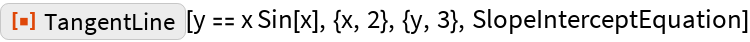Possible Issues

Vertical tangent lines (whose slope cannot be computed) are excluded from the results returned by TangentLine:

 In:=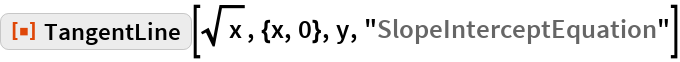Out=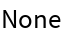This result is despite the fact that this expression has a vertical tangent line at the origin:

 In:=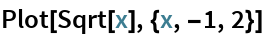Out=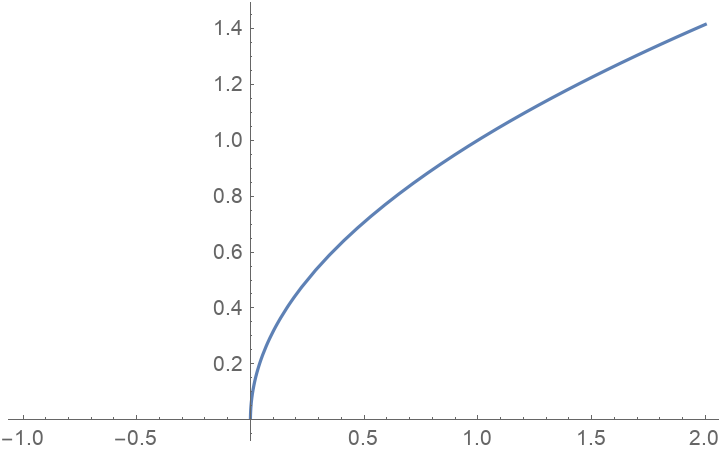If y is not specified, information on only one of the possible tangent lines at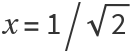is returned:

 In:=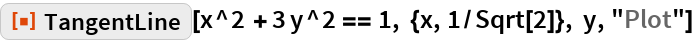Out=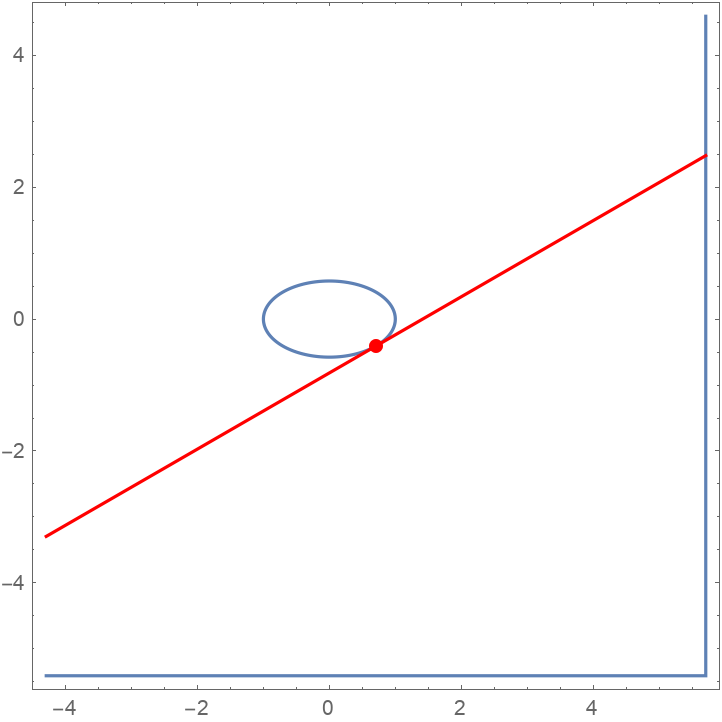To instead choose the other tangent line at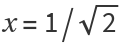, specify the corresponding value of y:

 In:=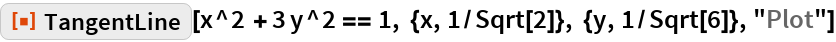Out=